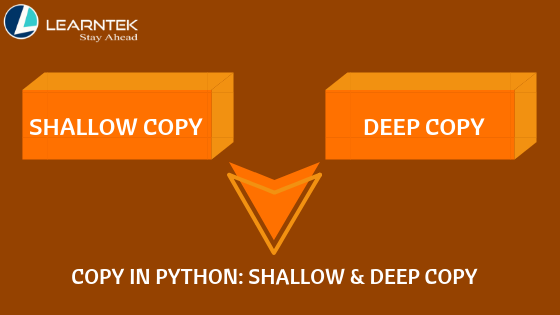# Shallow Copy and Deep Copy

Copy in python : Shallow Copy and Deep Copy

In Python programming, many times we need to make a copy of variable(s). In orders to make copy different methods are available in the Python programming.  Let’s take the example of the integer.

```>>> a = 10

>>> b = 10

>>> id(a)

140730625348928

>>> id(b)

140730625348928

>>>

>>> x = 257

>>> y = 257

>>> id(x)

2067355160528

>>> id(y)

2067355160432

>>>```

If an integer value is less than 256 then interpreter doesn’t make the copy, for a = 10 and b= 10 both the variables are referring to same memory allocation. But if the value exceeds the 256 then interpreter make a separate copy for each variable. See the figure below for more clarification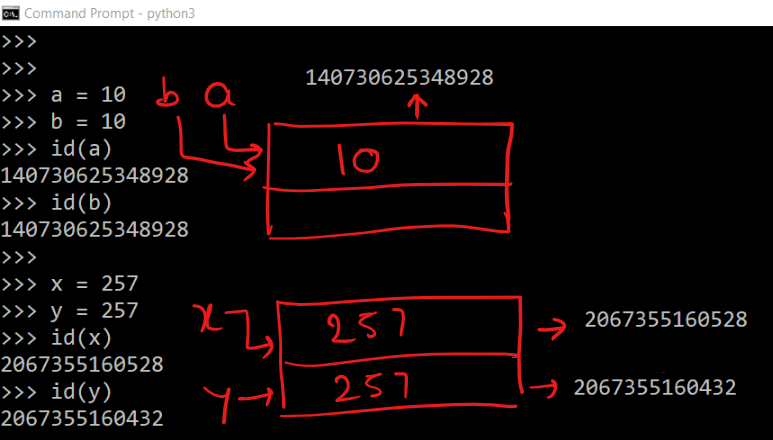Let us take the example of Python list.

See the following example

```>>> list1 = [1,2,3]

>>> list2 = [1,2,3]

>>> id(list1)

2067355168648

>>> id(list2)

2067353438600

>>>```

Addresses of both the lists are different. Let us create the copy of one variable.

```>>> list1 = [1,2,3]

>>> list3 = list1

>>>

>>> id(list1)

2067355168648

>>> id(list3)

2067355168648```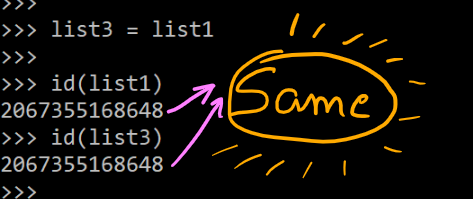It means both the variable are referring to same object or list.

Let see the different methods to create the copy of variable which refers to a list.

First method

```>>> list4 = list1[:]

>>> list4

[1, 2, 3]

>>> id(list4)

2067355181192

>>> id(list1)

2067355168648

>>>```

Second method

```>>>

>>> list5 = list(list1)

>>>

>>> id(list5)

2067355181640

>>>

>>> id(list1)

2067355168648

>>>```

You can check the addresses of list1 and list4, list1 and list5 are different.

Let us see one more example.

```>>> list1 = [1,2,["a","b"]]

>>>

>>> list2 = list1[:]

>>> list2

[1, 2, ['a', 'b']]

>>> id(list2)

2067355167944

>>> id(list1)

2067355168136

>>>```

Up to this level, nothing is surprising. Both the Python lists depicting different Python lists.

```>>> list1

['a', 'b']

>>> id(list1)

2067323314824

>>>

>>> id(list2)

2067323314824

>>>

>>> list2

['a', 'b']

>>>```

The above code is very surprising. It means list1 and list2 contain the same sub-list.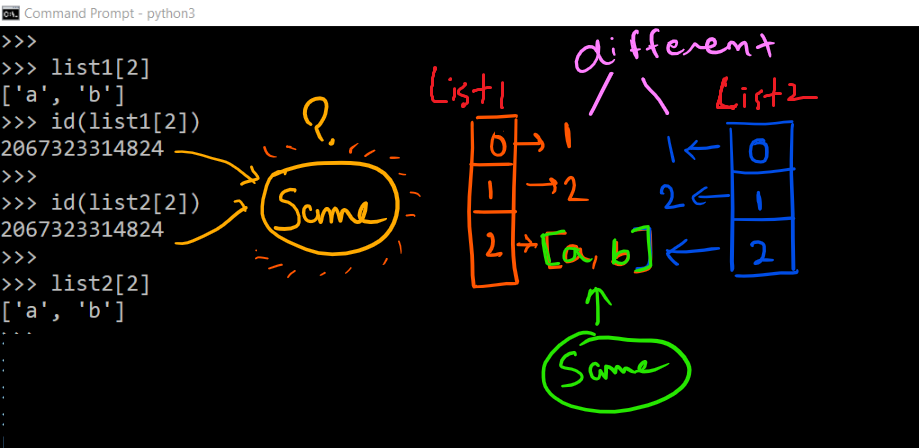This type of copy is called shallow copy. The inner sub-list address is same for the list1 and list2.

Let us append the inner sub-list.

```>>> list1 = [1,2,["a","b"]]

>>> list2 = list1

>>>

>>> list2 = list1[:]

>>> list2

[1, 2, ['a', 'b']]

>>> list1

[1, 2, ['a', 'b']]

>>>

>>> list1.append('c')

>>> list1

[1, 2, ['a', 'b', 'c']]

>>> list2

[1, 2, ['a', 'b', 'c']]

>>>```

You can see if the sub-list list1 is updated then the impact also reflected to the list2.

Learn Python  +  Advanced Python Training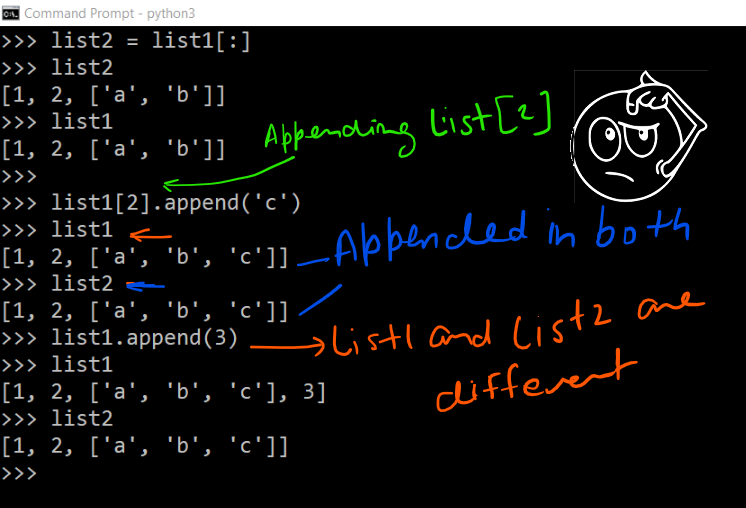If you use syntax list2 = list(list1) then this syntax would give you the same answer.

Deep Copy

Now, what is the solution of shallow copy problem? The solution is a deep copy which allows a complete copy of the list. In other words, It means first constructing a new collection object and then recursively populating it with copies of the child objects found in the original.

Fortunately, in Python, we have copy module to accomplish the task.

See the following series of commands

```>>> list1 = [1,2,["a","b"]]

>>> from copy import deepcopy

>>> list2 = deepcopy(list1)

>>> id(list1)

1724712551368

>>> id(list2)

1724744113928

>>>

>>> id(list1)

1724712051336

>>> id(list2)

1724713319304```

You can see in both the Python list, list1 and list2, the sub-list is at different addresses.

```>>> list2

['a', 'b']

>>> list2.append("c")

>>> list2

[1, 2, ['a', 'b', 'c']]

>>>

>>> list1

[1, 2, ['a', 'b']]

>>>```

If we append something in the sub-list of list2 then it does not reflect on the sub-list of list1.

Let us take a complex example

```>>> list1 = [1,2,("a","b",[1,2],3),4]

>>> list1

[1, 2, ('a', 'b', [1, 2], 3), 4]

>>> import copy

>>> list2 = copy.deepcopy(list1)

>>> list2

[1, 2, ('a', 'b', [1, 2], 3), 4]

>>> list1

[1, 2]

>>> list1.append(5)

>>> list1

[1, 2, ('a', 'b', [1, 2, 5], 3), 4]

>>> list2

[1, 2, ('a', 'b', [1, 2], 3), 4]

>>>```

We can say the deep copy is working fine.

Let us take the case of Dictionary. In the dictionary, with the help of method copy, we can produce a copy of the dictionary. Let us see an example.

```>>> dict1 = {1: 'a', 2: 'b', 3: ['C', 'D']}
>>>

>>> dict2 = dict1.copy()

>>>

>>> dict2

['C', 'D']

>>>

>>> dict2

{1: 'a', 2: 'b', 3: ['C', 'D']}

>>>

>>> dict2.append("E")

>>> dict2

{1: 'a', 2: 'b', 3: ['C', 'D', 'E']}

>>> dict1

{1: 'a', 2: 'b', 3: ['C', 'D', 'E']}

>>>

>>> id(dict1)

1724744113864

>>>

>>> id(dict2)

1724744113864

>>>```

So, what above series of command infers. See the following diagram.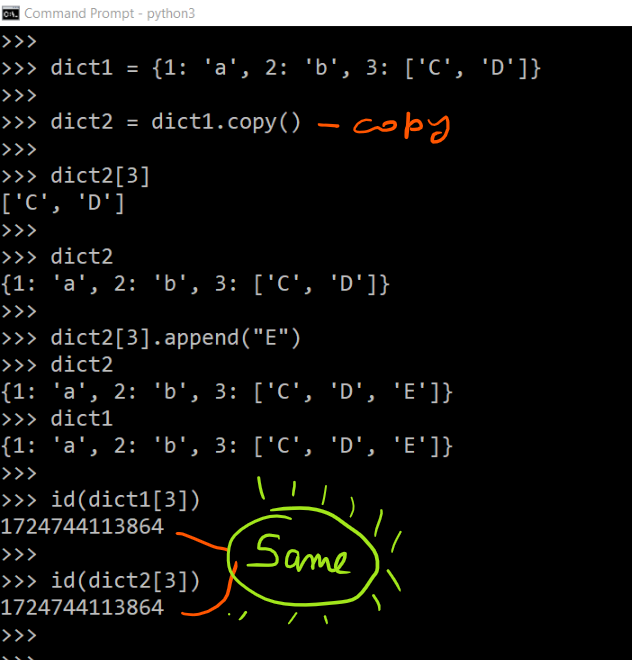It means the dict method copy also produce the shallow copy.

Let take the help of deep copy to produce to a deep copy.

```>>> dict3 = deepcopy(dict1)

>>> dict3

{1: 'a', 2: 'b', 3: ['C', 'D', 'E']}

>>> dict1.append("L")

>>>

>>> dict1

{1: 'a', 2: 'b', 3: ['C', 'D', 'E', 'L']}

>>>

>>> dict3

{1: 'a', 2: 'b', 3: ['C', 'D', 'E']}

>>>```

Means changes of dict1 are not reflecting dict3.

In the end we can conclude that

Making a shallow copy of an object won’t clone internal objects. Therefore, the copy is not fully independent of the original.

A deep copy of an object will recursively clone internal objects. The clone is fully independent of the original, but creating a deep copy is slower.